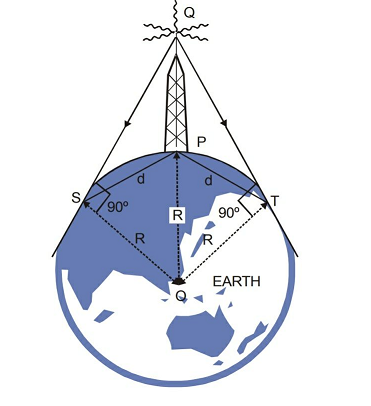Q

# Try this! A signal is to be transmitted through a wave of wavelength λ, using a linear antenna. The length l of the antenna and effective power radiated Peff will be given respectively as : (K is a constant of proportionality)

A signal is to be transmitted through a wave of wavelength λ, using a linear antenna. The length l of the antenna and effective power radiated Peff will be given respectively as : (K is a constant of proportionality)

• Option 1)• Option 2)• Option 3)• Option 4)842 Views

As we learnt in

Range of transmitting antenna -- wherein

height of antenna

Length of antenna = Comparable to

Power radiated by linear antenna inversly depends on the square wavelength and directly on the length of antenna.

Hence,

Correct option is 1.

Option 1)This is the correct option.

Option 2)This is an incorrect option.

Option 3)This is an incorrect option.

Option 4)This is an incorrect option.

Exams
Articles
Questions